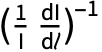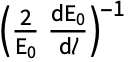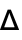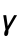# Zeeman Structure: Nonlinear Magneto-Optical Rotation

An atomic ground state withcan be polarized by the application of light resonant with a transition to an upper state. This polarization will precess about the direction of an applied magnetic field. The atomic polarization can then act back on the light to change the light's polarization, for example by rotating the polarization angle of the light, in a process known as nonlinear magneto-optical rotation (NMOR).

We define an atomic system consisting of a J=1 ground state and a J=0 upper state. We apply an x-polarized light field and a z-directed magnetic field.

 Define the atomic system.
 Remove explicit time dependence from the notation for the density-matrix elements.
 Here is what the density matrix looks like.
 The Hamiltonian for the system subject to the optical field, in terms of the "reduced" Rabi frequency ΩR and Larmor frequency ΩL.
 The level diagram for the system, showing the Zeeman splitting and the light coupling.
 Apply the rotating-wave approximation to the Hamiltonian.
 IntrinsicRelaxation and TransitRelaxation supply the relaxation matrices.
 OpticalRepopulation and TransitRepopulation supply the repopulation matrices.
 Here are the evolution equations.
 Observables supplies the fractional electric-field absorption, phase shift, polarization rotation, and change of ellipticity experienced by the light in terms of the density-matrix elements.

It is convenient to write the observables in terms of the absorption length in the atomic medium. The on-resonance, unsaturated absorption length is given by 0=-=-, withand ΩL equal to zero, and ΩR andapproaching zero.

 Find the absorption length by solving for the differential fractional change in electric field amplitude with zero detuning and magnetic field, and then letting the electric field amplitude and transit rate go to zero.
 The values of the observables per absorption length.

We can solve the equations numerically and plot the values of the observables as a function of various parameters. As a function of magnetic field, we see "nested features" in the line shapes: a narrow central resonance of width(the "transit effect"), and a wider resonance of width(the linear effect).

 Here are the absorption, phase shift, polarization rotation, and change of ellipticity as a function of Larmor frequency for particular values of the parameters.
 The absorption, phase shift, polarization rotation, and change of ellipticity as a function of light detuning.

We can also solve the equations analytically. The general solution is complicated, so it is helpful to look at specific cases. For example, if we are interested in the transit effect and wish to neglect the linear effect, we can make the assumptions γ, ΩL<<Γ.